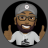# Add OHLC to lower timeframe chart?

B

#### bizzns

##### New member
Hi all,

Is there a way to have Daily OHLC lines plot on a short time frame chart that I use for a trigger? For instance, If I go and plot out my daily OHLC lines then I can see them on my short term (trigger chart). However, when I jump to a different ticker I have to re-evaluate and spend time plotting which takes away valuable time during trading. Any suggestions on how this can be done.#### inthefutures

##### Active member
add a aggregate time period to your code line with the time period of your trigger chart

B

#### bizzns

##### New member
I can't even find a study that plots OHLC on my charts to begin with... I wish I knew something about coding... unfortunately, I'm completely inept on the subject.#### inthefutures

##### Active member
so you want yesterday's ohlc ploted on today's say 5 minute chart?

B

#### bizzns

##### New member
Well basically I’d like to have past few days (ie 10 days) plotted on my short term chart. I actually use a 200 tick chart for my trigger chart. Also use 1min and 2 min... they seem to correlate fairly close.

This is for a quick in/out scalping sort of trades.

Last edited:#### inthefutures

##### Active member
Code:
``````[CODE]#April 11th 2020
#last 10 days OHLC
#Inthefutures

def agg = AggregationPeriod.DAY;

plot open = open(period = agg);
plot high = high(period = agg);
plot low = low(period = agg);
plot close = close(period = agg);

plot open2 = open(period = agg);
plot high2 = high(period = agg);
plot low2 = low(period = agg);
plot close2 = close(period = agg);

plot open3 = open(period = agg);
plot high3 = high(period = agg);
plot low3 = low(period = agg);
plot close3 = close(period = agg);

plot open4 = open(period = agg);
plot high4 = high(period = agg);
plot low4 = low(period = agg);
plot close4 = close(period = agg);

plot open5 = open(period = agg);
plot high5 = high(period = agg);
plot low5 = low(period = agg);
plot close5 = close(period = agg);

plot open6 = open(period = agg);
plot high6 = high(period = agg);
plot low6 = low(period = agg);
plot close6 = close(period = agg);

plot open7 = open(period = agg);
plot high77 = high(period = agg);
plot low7 = low(period = agg);
plot close7 = close(period = agg);

plot open8 = open(period = agg);
plot high8 = high(period = agg);
plot low8 = low(period = agg);
plot close8 = close(period = agg);

plot open9 = open(period = agg);
plot high9 = high(period = agg);
plot low9 = low(period = agg);
plot close9 = close(period = agg);

plot open10 = open(period = agg);
plot high10 = high(period = agg);
plot low10 = low(period = agg);
plot close10 = close(period = agg);``````
[/CODE]

•marcusrg3
B

#### bizzns

##### New member
Appreciate you putting your time in for this. However, its not plotting. The name of the indicator is highlighted red after I add it to my studies. Not sure if that means its missing something?#### inthefutures

##### Active member
you must not have copied the # before the words

•bizzns
B

#### bizzns

##### New member
yes! I appreciate this so much. It'll save me loads of time. thank you so much.

B

#### bizzns

##### New member
Hey buddy. You wouldnt be familiar with Tradestation code system by chance would you? TOS has been very unreliable lately so i have an alternative but this code helps so much...#### inthefutures

##### Active member
no do not know TSCan you add "AddChartBubble"to a Lower Chart? Questions 2